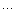## F#探险之旅（七）：在F#中进行单元测试

2008-11-18 13:42  Anders Cui  阅读(2906)  评论(0编辑  收藏  举报F# Code - mathHelper的签名#lightmodule MyFsLib.MathHelper/// 获取一个浮点数的平方值val square: float -> float/// 获取一个浮点数的立方值val cube: float -> float/// 判断一个整数是否为偶数val isEven: int -> bool/// 判断一个整数是否为奇数val isOdd: int -> bool/// 获取不大于指定正整数的质数数组val generatePrimes: int -> int arrayF# Code - mathHelper的实现#lightmodule MyFsLib.MathHelperopen Systemlet pow x y = Math.Pow(x, y)let square x = pow x 2.0let cube x = pow x 3.0let isEven x = x % 2 = 0let isOdd x = x % 2 = 1// Eratosthenes筛法let generatePrimes n =    match n with    | _ when n < 2 -> [||]    | _ ->        // Init sieve.        let sieve = [| for i in 0 .. n do yield true |]               let isPrime index = sieve.[index]               // Check it.        let upperBound = Convert.ToInt32(Math.Sqrt((float)n))        for i = 2 to upperBound do            if isPrime i then                for j in [i * 2 .. i .. sieve.Length - 1] do                    sieve.[j] <- false                           let mutable count = 0        for i = 2 to sieve.Length - 1 do            if isPrime i then                count <- count + 1                       let primes = Array.create count 0        let mutable index = 0        for i = 2 to sieve.Length - 1 do            if isPrime i then                primes.[index] <- i                index <- index + 1                       primesF# Code - NUnit tester#lightnamespace NUnitTesteropen NUnit.Frameworkopen MyFsLib[<TestFixture>]type TestCases = class    new() = {}       [<Test>]    member this.TestSquare() =        Assert.AreEqual(0, MathHelper.square(0.0))        Assert.AreEqual(4, MathHelper.square(2.0))           [<Test>]    member this.TestGeneratePrimes() =        let primesLessThan2 = MathHelper.generatePrimes(1)        CollectionAssert.IsEmpty(primesLessThan2)               let primesNotGreaterThan2 = MathHelper.generatePrimes(2)        CollectionAssert.IsNotEmpty(primesNotGreaterThan2)        CollectionAssert.Contains(primesNotGreaterThan2, 2)        Assert.AreEqual(1, primesNotGreaterThan2.Length)               let primesNotGreaterThan10 = MathHelper.generatePrimes(10)        CollectionAssert.IsNotEmpty(primesNotGreaterThan10)        CollectionAssert.Contains(primesNotGreaterThan10, 7)        Assert.AreEqual(4, primesNotGreaterThan10.Length)           // Other testcasesendFsUnit是一个Specification测试框架。它的目标是将单元测试和行为（函数）的规格尽量简化，并以函数式的风格代替命令式风格的测试代码。

Specification可以翻译为规格说明，就是说测试代码实际上是对待测代码的一条条规格说明。比如对函数square，它求一个数的平方，那么一条规格可以是：”square(2) should equal 4”。好了，惊喜就要来了：F# Code - FsUnit tester#lightopen FsUnitopen MyFsLiblet squareSpecs =    specs "Test square" [        spec "square(0) should equal 0"             (MathHelper.square(0.0) |> should equal 0.0) // Pass        spec "square(2) should equal 2"             (MathHelper.square(2.0) |> should equal 2.0) // Fail    ]    let generatePrimes1Specs =    specs "Test generatePrimes" [        spec "generatePrimes(1).Length should equal 0"            (MathHelper.generatePrimes(1).Length |> should equal 0)    ]    let generatePrimes2Specs =    specs "Test generatePrimes" [        spec "generatePrimes(2).Length should equal 1"            (MathHelper.generatePrimes(2).Length |> should equal 1)        spec "generatePrimes(2) should contain 2"            (MathHelper.generatePrimes(2) |> should contain 2)    ]    let generatePrimes10Specs =    specs "Test generatePrimes" [        spec "generatePrimes(10).Length should equal 4"            (MathHelper.generatePrimes(10).Length |> should equal 4)        spec "generatePrimes(10) should contain 7"            (MathHelper.generatePrimes(10) |> should contain 7)        spec "generatePrimes(10) should not contain 9"            (MathHelper.generatePrimes(10) |> should not' (contain 9))    ]    printfn "%s" (Results.summary())（要了解本人所写的其它F#随笔请查看 F#系列随笔索引

《Foundations of F#》 by Robert Pickering